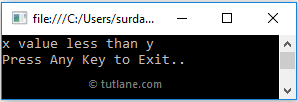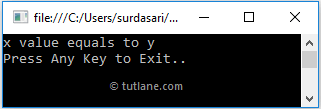# Visual Basic Ternary Operator

In Visual Basic, Ternary Operator is a decision-making operator, and it is an alternative for the if…else statement in Visual Basic programming language.

Using Ternary Operator, we can replace the multiple lines of if…else statement code into a single line in Visual Basic. The Ternary operator will help you execute the statements based on the defined conditions using the comma (,) separated operator.

## Syntax of Visual Basic Ternary Operator

In Visual Basic, the Ternary Operator will always work with three operands. Following is the syntax of defining the Ternary Operator in Visual Basic programming language.

If((condition_expression), first_expression, second_expression)

If you observe the above Ternary Operator syntax, the conditional operator will return only one value from the defined expressions, i.e., first_expression or second_expression, based on the value of a condition.

In Visual Basic, the Ternary Operator will work as follow.

• In Ternary Operator, the condition expression must be evaluated as true or false. If the condition is true, the first_expression result is returned by the ternary operator.
• If the condition is false, then the second_expression result is returned by the operator.

As said earlier, the Ternary Operator is an alternative of if…else statement in Visual Basic programming language. For example, we can replace the following if…else statement with Ternary Operator as shown below.

Dim x As Integer = 5, y As Integer = 20
Dim result As String
If x > y Then
result = "x greater than y"
Else
result = "x less than y"
End If
result = If((x > y), "x greater than y", "x less than y")

If you observe the above example, we simplified the if…else condition by replacing the multiple lines of if…else statement code with Ternary Operator in Visual Basic programming language.

Now, we will see the complete example of a Ternary operator in Visual Basic programming language.

## Visual Basic Ternary Operator Example

Following is the example of using Ternary Operator in Visual Basic programming language.

Module Module1
Sub Main()
Dim x As Integer = 5, y As Integer = 20
Dim result As String
result = If((x > y), "x value greater than y", "x value less than y")
Console.WriteLine(result)
Console.WriteLine("Press Enter Key to Exit..")
End Sub
End Module

If you observe the above example, we used the Ternary Operator to evaluate an expression (x > y) and to show the result based on our requirements.

When we execute the above Visual Basic program, we will get the result as shown below.This is how we can use the Ternary Operator as a substitute for if…else statement in Visual Basic programming language.

## Visual Basic Nested Ternary Operator

In Visual Basic, we can create a Nested Ternary Operator by including the multiple conditional expressions as a second or third part of the ternary operator. These nested ternary operators will help us replace the if…else if statements in Visual Basic programming language.

Following is the example of replacing the if…else if statement with the nested ternary operator in Visual Basic programming language.

Dim x As Integer = 20, y As Integer = 20
Dim result As String
If x > y Then
result = "x value greater than y"
ElseIf x < y Then
result = "x value less than y"
Else
result = "x value equals to y"
End If
result = If((x > y), "x value greater than y", If((x < y), "x value less than y", "x value equals to y"))

If you observe the above code, we can replace the multiple lines of if…else if code with a single line of the nested ternary operator based on our requirements.

In Visual Basic, the conditional operator is a right-associative, so the expression a, b, c, d, e; evaluated as a, b, (c, d, e), not as (a, b, c), d, e.

## Visual Basic Nested Ternary Operator Example

Following is the example of defining the nested ternary operator in Visual Basic programming language.

Module Module1
Sub Main()
Dim x As Integer = 20, y As Integer = 20
Dim result As String
result = If((x > y), "x value greater than y", If((x < y), "x value less than y", "x value equals to y"))
Console.WriteLine(result)
Console.WriteLine("Press Enter Key to Exit..")
End Sub
End Module

When we execute the above Visual Basic program, we will get the result as shown below.This is how we can implement a nested ternary operator in Visual Basic programming language to replace if…else if statements based on our requirements.